# Geometric Data Types

PostgreSQL supports six data types that represent two-dimensional geometric objects. The most basic geometric data type is the POINT as you might expect, a POINT represents a point within a two-dimensional plane.

A POINT is composed of an x-coordinate and a y-coordinateeach coordinate is a DOUBLE PRECISION number.

The LSEG data type represents a two-dimensional line segment. When you create a LSEG value, you specify two pointsthe starting POINT and the ending POINT.

A BOX value is used to define a rectanglethe two points that define a box specify opposite corners.

A PATH is a collection of an arbitrary number of POINT s that are connected. A PATH can specify either a closed path or an open path. In a closed path, the beginning and ending points are considered to be connected, and in an open path, the first and last points are not connected. PostgreSQL provides two functions to force a PATH to be either open or closed: POPEN() and PCLOSE(). You can also specify whether a PATH is open or closed using special literal syntax (described later).

A POLYGON is similar to a closed PATH. The difference between the two types is in the supporting functions.

A center POINT and a (DOUBLE PRECISION) floating-point radius represent a CIRCLE.

Table 2.18 summarizes the geometric data types.

Table 2.18. Geometric Data Types

Type

Meaning

Defined By

POINT

2D point on a plane

x- and y-coordinates

LSEG

Line segment

Two points

BOX

Rectangle

Two points

PATH

Open or closed path

n points

POLYGON

Polygon

n points

CIRCLE

Circle

Syntax for Literal Values

When you enter a value for geometric data type, keep in mind that you are working with a list of two-dimensional points (except in the case of a CIRCLE, where you are working with a POINT and a radius).

A single POINT can be entered in either of the following two forms:

```'( x, y )'
' x, y '
```

The LSEG and BOX types are constructed from a pair of POINT s. You can enter a pair of POINT s in any of the following formats:

```'(( x1, y1 ), ( x2, y2 ))'
'( x1, y1 ), ( x2, y2 )'
'x1, y1, x2, y2'
```

The PATH and POLYGON types are constructed from a list of one or more POINT s. Any of the following forms is acceptable for a PATH or POLYGON literal:

```'(( x1, y1 ), ..., ( xn, yn ))'
'( x1, y1 ), ..., ( xn, yn )'
'( x1, y1, ..., xn, yn )'
'x1, y1, ..., xn, yn'
```

You can also use the syntax '[( x1, y1 ), ..., ( xn, yn )]' to enter a PATH literal. A PATH entered in this form is considered to be an open PATH.

A CIRCLE is described by a central point and a floating point radius. You can enter a CIRCLE in any of the following forms:

```'< ( x, y ), r >'
'(( x, y ), r )'
'( x, y ), r'
'x, y, r'
```

Notice that the surrounding single quotes are required around all geometric literalsin other words, geometric literals are entered as string literals. If you want to create a geometric value from individual components, you will have to use a geometric conversion function. For example, if you want to create a POINT value from the results of some computation, you would use:

```POINT( 4, 3*height )
```

The POINT( DOUBLE PRECISION x, DOUBLE PRECISION y ) function creates a POINT value from two DOUBLE PRECISION values. There are similar functions that you can use to create any geometric type starting from individual components. Table 2.19 lists the conversion functions for geometric types.

Table 2.19. Type Conversion Operators for the Geometric Data Types

Result Type

Meaning

POINT

POINT( DOUBLE PRECISION x, DOUBLE PRECISION y )

LSEG

LSEG( POINT p1, POINT p2 )

BOX

BOX( POINT p1, POINT p2 )

PATH

PATH( POLYGON poly )

POLYGON

POLYGON( PATH path )

POLYGON( BOX b ) yields a 12-point polygon

POLYGON( CIRCLE c ) yields a 12-point polygon

POLYGON( INTEGER n, CIRCLE c ) yields an n point polygon

CIRCLE

CIRCLE( BOX b )

CIRCLE( POINT radius, DOUBLE PRECISION point )

Sizes and Valid Values

Table 2.20 lists the size of each geometric data type.

Table 2.20. Geographic Data Type Storage Requirements

Type

Size (in bytes)

POINT

16 (2 * sizeof DOUBLE PRECISION)

LSEG

32 (2 * sizeof POINT)

BOX

32 (2 * sizeof POINT)

PATH

4+(32*number of points)

POLYGON

4+(32*number of points)

CIRCLE

24 (sizeof POINT + sizeof DOUBLE PRECISION)

 The size of a PATH or POLYGON is equal to 4 + ( size of LSEG * number of segments).

Supported Operators

PostgreSQL features a large collection of operators that work with the geometric data types. I've divided the geometric operators into two broad categories (transformation and proximity) to make it a little easier to talk about them.

Using the transformation operators, you can translate, rotate, and scale geometric objects. The + and - operators translate a geometric object to a new location. Consider Figure 2.1, which shows a BOX defined as BOX( POINT( 3,5 ), POINT( 1,2 )).

Figure 2.1. BOX( POINT( 3,5 ), POINT( 1,2 )).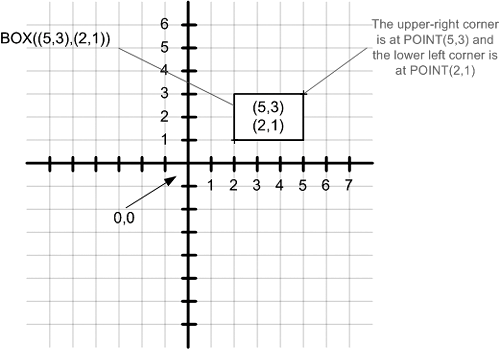If you use the + operator to add the POINT( 2,1 ) to this BOX, you end up with the object shown in Figure 2.2.

Figure 2.2. Geometric translation.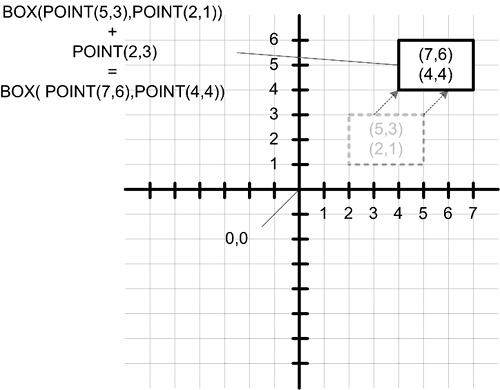You can see that the x-coordinate of the POINT is added to each of the x-coordinates in the BOX, and the y-coordinate of the POINT is added to the y-coordinates in the BOX. The - operator works in a similar fashion: the x-coordinate of the POINT is subtracted from the x-coordinates of the BOX, and the y-coordinate of the POINT is subtracted from each y-coordinate in the BOX.

Using the + and - operators, you can move a POINT, BOX, PATH, or CIRCLE to a new location. In each case, the x-coordinate in the second operand (a POINT), is added or subtracted from each x-coordinate in the first operand, and the y-coordinate in the second operand is added or subtracted from each y-coordinate in the first operand.

The multiplication and division operators ( * and /) are used to scale and rotate. The multiplication and division operators treat the operands as points in the complex plane. Let's look at some examples.

Figure 2.3 shows the result of multiplying BOX(POINT(3,2),POINT(1,1)) by POINT(2,0).

Figure 2.3. Point multiplication-scaling by a positive value.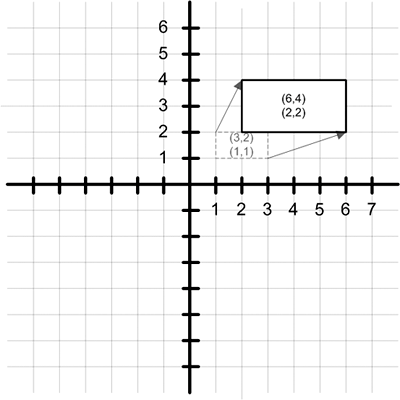You can see that each coordinate in the original box is multiplied by the x-coordinate of the point, resulting in BOX(POINT(6,4),POINT(2,2)). If you had multiplied the box by POINT(0.5,0), you would have ended up with BOX(POINT(1.5,1),POINT(0.5,0.5)). So the effect of multiplying an object by POINT(x,0) is that each coordinate in the object moves away from the origin by a factor x. If x is negative, the coordinates move to the other side of the origin, as shown in Figure 2.4.

Figure 2.4. Point multiplication-scaling by a negative value.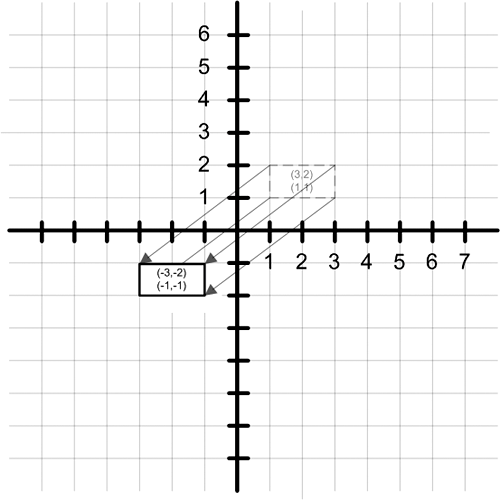The x-coordinate controls scaling. The y-coordinate controls rotation. When you multiply any given geometric object by POINT(0,y), each point in the object is rotated around the origin. When y is equal to 1, each point is rotated counterclockwise by 90° about the origin. When y is equal to 1, each point is rotated 90° about the origin (or 270°). When you rotate a point without scaling, the length of the line segment drawn between the point and origin remains constant, as shown in Figure 2.5.

Figure 2.5. Point multiplication-rotation.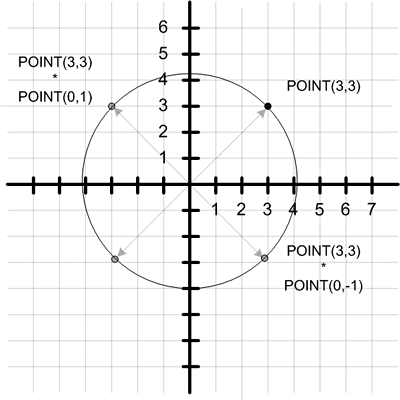You can combine rotation and scaling into the same operation by specifying non-zero values for both the x- and y-coordinates. For more information on using complex numbers to represent geometric points, see http://www.clarku.edu/~djoyce/complex.

Table 2.21 shows the valid combinations for geometric types and geometric operators.

Table 2.21. Transformation Operators for the Geometric Types

Data Types

Valid Operators (q)

POINT q POINT

* + - /

BOX q POINT

* + - /

PATH q POINT

* + - /

CIRCLE q POINT

* + - /

The proximity operators allow you to determine the spatial relationships between two geometric objects.

First, let's look at the three containment operators. The ~ operator evaluates to TRUE if the left operand contains the right operand. The @ operator evaluates to TRUE if the left operand is contained within the right operand. The ~= returns trUE if the left operand is the same as the right operandtwo geographic objects are considered identical if the points that define the objects are identical (two circles are considered identical if the radii and center points are the same).

The next two operators are used to determine the distance between two geometric objects.

The ## operator returns the closest point between two objects. You can use the ## operator with the following operand types shown in Table 2.22.

Table 2.22. Closest-Point Operators

Operator

Description

LSEG a ## BOX b

Returns the point in BOX b that is closest to LSEG a

LSEG a ## LSEG b

Returns the point in LSEG b that is closest to LSEG a

POINT a ## BOX b

Returns the point in BOX b that is closest to POINT a

POINT a ## LSEG b

Returns the point in LSEG b that is closest to POINT a

The distance (<->) operator returns (as a DOUBLE PRECISION number) the distance between two geometric objects. You can use the distance operator with the operand types in Table 2.23.

Table 2.23. Distance Operators

Operator

Description (or Formula)

BOX a <-> BOX b

(@@ BOX a) <-> (@@ BOX b)

CIRCLE a <-> CIRCLE b

(@@ CIRCLE a) <-> (@@ CIRCLE b)

-

CIRCLE a <-> POLYGON b

-0 if any point in POLYGON b is inside CIRCLE a otherwise, distance between center of CIRCLE a and closest point in POLYGON b

LSEG a <-> BOX b

(LSEG ## BOX) <-> (LSEG ## (LSEG ## BOX))

LSEG a <-> LSEG b

Distance between closest points (0 if LSEG a intersects LSEG b)

PATH a <-> PATH b

Distance between closest points

POINT a <-> BOX b

POINT a <-> (POINT a ## BOX b )

POINT a <-> CIRCLE b

POINT a <-> ((@@ CIRCLE b) - CIRCLE b radius)

POINT a <-> LSEG b

POINT a <-> (POINT a ## LSEG b)

POINT a <-> PATH b

Distance between POINT a and closest points

POINT a <-> POINT b

-SQRT(( POINT a.x - POINT b.x ) 2 + (POINT a.y - POINT b.y ) 2 )

Next, you can determine the spatial relationships between two objects using the left-of (<<), right-of(>>), below (<^), and above (>^) operators.

There are three overlap operators. && evaluates to trUE if the left operand overlaps the right operand. The &> operator evaluates to trUE if the leftmost point in the first operand is left of the rightmost point in the second operand. The &< evaluates to TRUE if the rightmost point in the first operand is right of the leftmost point in the second operand.

The intersection operator (#) returns the intersecting points of two objects. You can find the intersection of two BOX es, or the intersection of two LSEG s. The intersection of two BOX es evaluates to a BOX. The intersection of two LSEG s evaluates to a single POINT.

Finally, the q# operator evaluates to trUE if the first operand intersects with or overlaps the second operand.

The final set of geometric operators determines the relationship between a line segment and an axis, or the relationship between two line segments.

The q- operator evaluates to trUE if the given line segment is horizontal (that is, parallel to the x-axis). The q| operator evaluates to trUE if the given line segment is vertical (that is, parallel to the y-axis). When you use the q- and q| operators with a line segment, they function as prefix unary operators. You can also use the q- and q| operators as infix binary operators (meaning that the operator appears between two values), in which case they operate as if you specified two points on a line segment.

The q-| operator evaluates to trUE if the two operands are perpendicular. The q|| operator evaluates to trUE if the two operands are parallel. The perpendicular and parallel operators can be used only with values of type LSEG.

The final geometric operator (@@) returns the center point of an LSEG, PATH, BOX, POLYGON, or CIRCLE.

Tables 2.24 summarizes the proximity operators.

Table 2.24. Proximity Operators for the Geometric Types

Data Types

Valid Operators (q)

POINT q POINT

<-> << <^ >> >^ q- q| @

POINT q LSEG

## <-> @

POINT q BOX

## <-> @

POINT q PATH

<-> @

POINT q POLYGON

@

POINT q CIRCLE

<-> @

LSEG q LSEG

# ## < <-> <= <> = > >= q# q-| q||

LSEG q BOX

## <-> q# @

BOX q POINT

* + - /

BOX q BOX

# && &< &> < <-> << <= <^ = > >= >> >^ q# @ ~ ~=

PATH q POINT

* + - / ~

PATH q PATH

+ < <-> <= = > >= q#

POLYGON q POINT

~

POLYGON q POLYGON

&& &< &> <-> >> << @ ~ ~=

CIRCLE q POINT

* + - / ~

CIRCLE q POLYGON

<->

CIRCLE q CIRCLE

&& &< &> > <-> << <= <> <^ = > >= >> >^ @ ~ ~=

Table 2.25 summarizes the names of the proximity operators for geometric types.

Table 2.25. Geometric Proximity Operator Names

Data Types

Valid Operators (q)

#

Intersection or point count(for polygons)

##

Point of closest proximity

<->

Distance between

<<

Left of q

>>

Right of q

<^

Below q

>^

Above q

&&

Overlaps

&>

Overlaps to left

&<

Overlaps to right

q#

Intersects or overlaps

@

Contained in

~

Contains

~=

Same as

q-

Horizontal

q|

Vertical

q-|

Perpendicular

q||

Parallel

@@

CenterPostgreSQL(c) The comprehensive guide to building, programming, and administering PostgreSQL databases
ISBN: 735712573
EAN: N/A
Year: 2004
Pages: 261